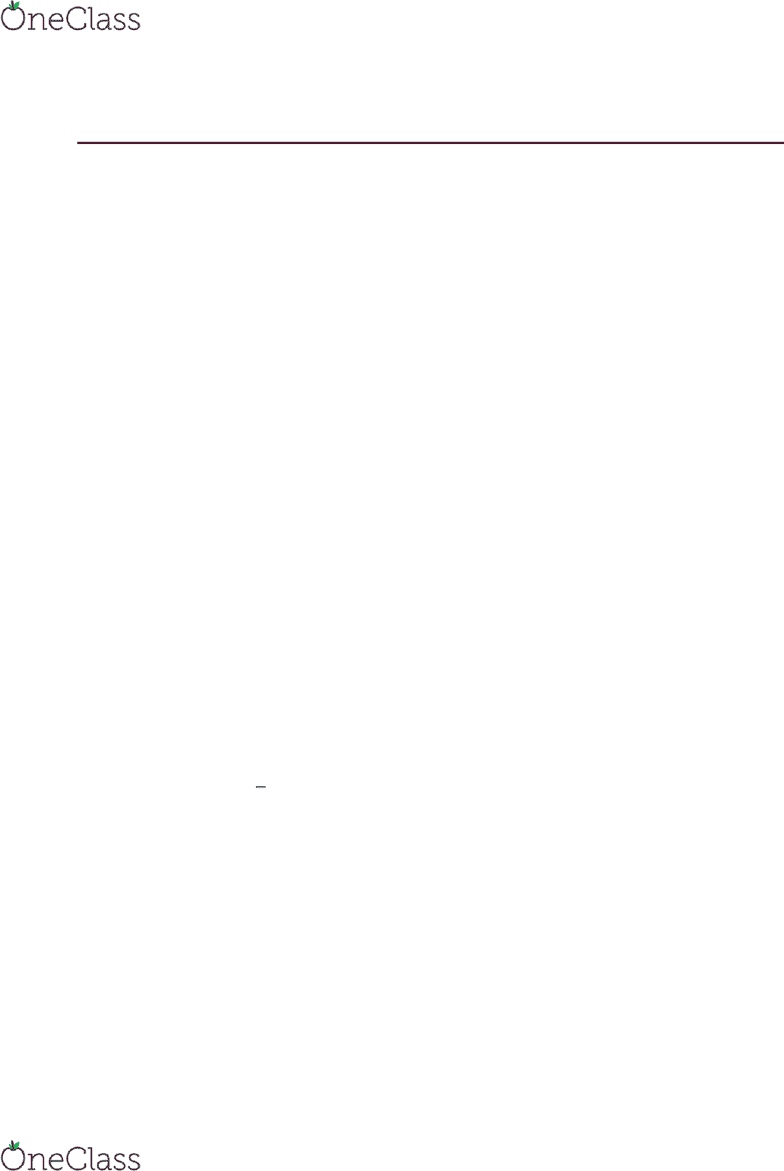Class Notes (1,100,000)
US (490,000)
TU (2,000)
CHEM (50)
Lecture 7

# CHEM 131 Lecture Notes - Lecture 7: Single Displacement Reaction, Molar Mass, Chemical Formula

This preview shows half of the first page. to view the full 3 pages of the document.Chem 131 9/22
Example
1. The compound serotonin contains carbon (68.2% by mass), hydrogen
(6.86%), nitrogen (15.9%), and oxygen (9.08%). The molar mass of
serotonin is 176 g/mol. Determine the molecular formula of serotonin
a. Carbon 68.2/12.010 = 5.679/0.568 = 10
b. Hydrogen 6.86/1.007 = 6.812/0.568 = 12
c. Nitrogen 15.9/14.006 = 1.135/0.568 = 2
d. Oxygen 9.08/15.999 = 0.568/0.568 = 1
e. So the equation is
i. C10H12N2O
Balancing Chemical Equations
2. 5 steps, to be posted onto blackboard
3. Example
a. Ethane reacts with oxygen to form carbon dioxide and water
b. C2H6 + O2 CO2 + H2O
c. C2H6 + (7
) O2 •CO2 + •H2O
i. C2H6 + O7 C2O4 + H6O3
4. Example
a. C3H8 + O2 CO2 + H2O
b. C3H8 + •O2 • CO2 + •H2O
i. C3H8 + O10 C3O6 + H8O4
5. Example
find more resources at oneclass.com
find more resources at oneclass.com